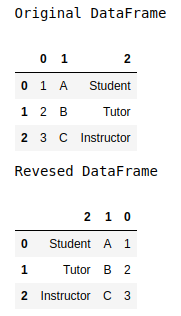# How to reverse the column order of the Pandas DataFrame?

Sometimes when working with DataFrames we might want to change or reverse the order of the column of the dataframe. In this article, let’s see how to reverse the order of the columns of a dataframe. This can be achieved in two ways –

Method 1: The sequence of columns appearing in the dataframe can be reversed by using the attribute.columns[::-1] on the corresponding dataframe. It accesses the columns from the end and outer dataframe[…] reindexes the dataframe using this new sequence provided.

Example:

## Python3

 `# importing required modules` `import` `pandas as pd`     `dataframe ``=` `pd.DataFrame([[``1``, ``'A'``, ``"Student"``], ` `                          ``[``2``, ``'B'``, ``"Tutor"``], ` `                          ``[``3``, ``'C'``, ``"Instructor"``]])`   `print``(``"Original DataFrame"``)` `display(dataframe)`   `# reversing the dataframe` `print``(``"Reversed DataFrame"``)` `display(dataframe[dataframe.columns[::``-``1``]])`

Output:Method 2: iloc indexer can also be used to reverse the column order of the data frame, using the syntax iloc[:, ::-1] on the specified dataframe. The contents are not preserved in the original dataframe.

## Python3

 `# importing required modules` `import` `pandas as pd`     `dataframe ``=` `pd.DataFrame([[``1``, ``'A'``, ``"Student"``], ` `                          ``[``2``, ``'B'``, ``"Tutor"``], ` `                          ``[``3``, ``'C'``, ``"Instructor"``]])`   `print``(``"Original DataFrame"``)` `display(dataframe)`   `# reversing the dataframe` `print``(``"Reversed DataFrame"``)` `display(dataframe.iloc[:, ::``-``1``])`

Output:Whether you're preparing for your first job interview or aiming to upskill in this ever-evolving tech landscape, GeeksforGeeks Courses are your key to success. We provide top-quality content at affordable prices, all geared towards accelerating your growth in a time-bound manner. Join the millions we've already empowered, and we're here to do the same for you. Don't miss out - check it out now!

Previous
Next